# How to Create Pie Charts in R [Real-life Project]

A pie chart is a data visualization type representing data in a circular format. Each slice of the circle represents a proportion of the whole, making pie charts especially helpful for understanding parts-to-whole relationships.

## Syntax

``pie(x, labels, radius, main, col, clockwise)``

## Parameters

1. x: It shows the positive input values for your chart.
2. labels: It shows the heading or description of each slice of your chart.
3. radius: It shows the radius of the circle regarding your chart. Its value must be between -1 and +1.
4. main: This suggests the title of your graph.
5. col: It is used for showing the color palette.
6. clockwise: It is used to set a value to draw the pie chart slices clockwise or anti-clockwise.

## Creating a pie chart from a dataset

To create pie charts in R, use the “pie()” method.

For this project, we will use Kaggle’s California Housing Price dataset.

We will use RStudio to display charts, so if you have not installed it, I highly recommend following this guide: How to Install RStudio.

Here is the step-by-step guide:

### Step 1: Install the necessary libraries

Install “tidyverse” and “plotrix” libraries using this code:

``````install.packages("tidyverse")

install.packages("plotrix")``````

The plotrix library can be used to construct 3D pie charts.

### Step 2: Import and load the dataset

To import and load the csv dataset into the data frame, use the built-in “read_csv()” method.

``````data <- read_csv("DataSets/california_housing.csv")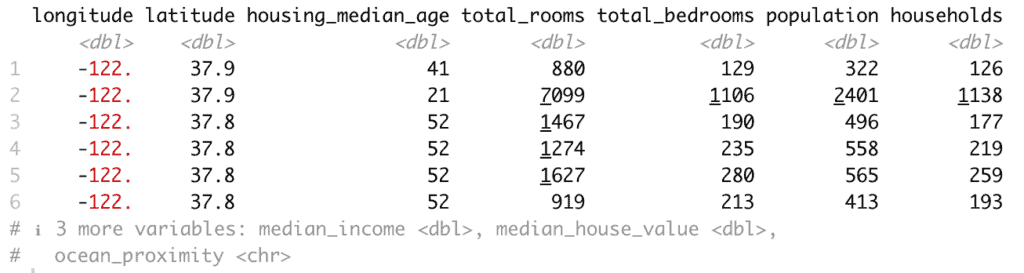We used the head() function to get an overview of a dataset, as seen in the screenshot above.

### Step 3: Visualize the pie chart

Let’s go ahead use the pie() function with the data we have on ocean_proximity.

``````data <- read_csv("DataSets/california_housing.csv")

# Compute the data for pie chart
data_summary <- data %>%
group_by(ocean_proximity) %>%
summarize(count = n())

# Custom colors
colors <- c("blue", "green", "lightblue", "orange", "yellow")

# Create a pie chart using base R
pie(data_summary\$count, labels = data_summary\$ocean_proximity,
main="Distribution of Houses Based on Proximity to Ocean in California",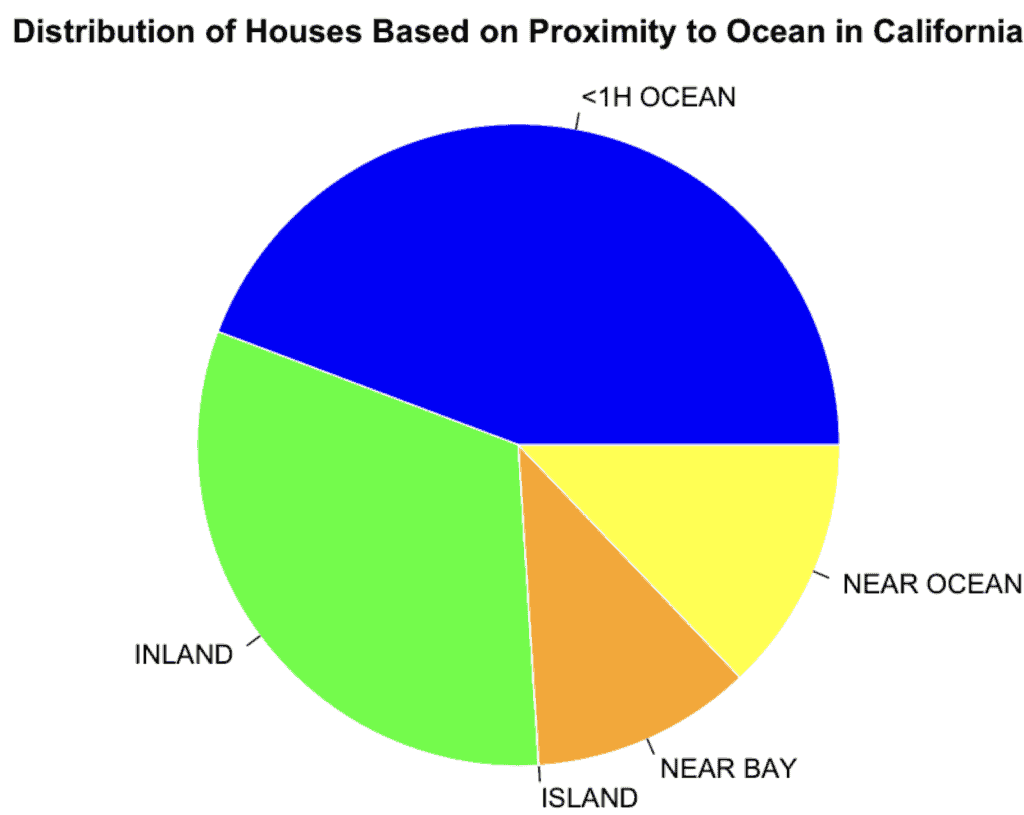Let me explain what is happening from the chart:

1. data_summary\$count provides the counts for each category.
2. labels define the labels for each slice.
3. main sets the title of the pie chart.
4. col sets the color for each slice.
5. border defines the border color of the slices.

## Changing the Pie Chart Title and Colors

You can customize the title and colors based on the ocean_proximity column in the dataset.

Here’s how you can do it:

### Calculate the data for the pie chart:

``data_summary <- table(data\$ocean_proximity)``

### Define custom colors:

``````colors <- c("<1H OCEAN" = "blue", "INLAND" = "green",
"NEAR OCEAN" = "lightblue", "NEAR BAY" = "teal", "ISLAND" = "yellow")``````

### Constructing a chart

``````data <- read_csv("DataSets/california_housing.csv")

# Compute the data for pie chart
data_summary <- table(data\$ocean_proximity)

colors <- c("<1H OCEAN" = "beige", "INLAND" = "green",
"NEAR OCEAN" = "blue", "NEAR BAY" = "violet", "ISLAND" = "red")

pie(data_summary,
labels = names(data_summary),
main="Distribution of Houses Based on Proximity to Ocean in California",
col=colors,
border="white",
``````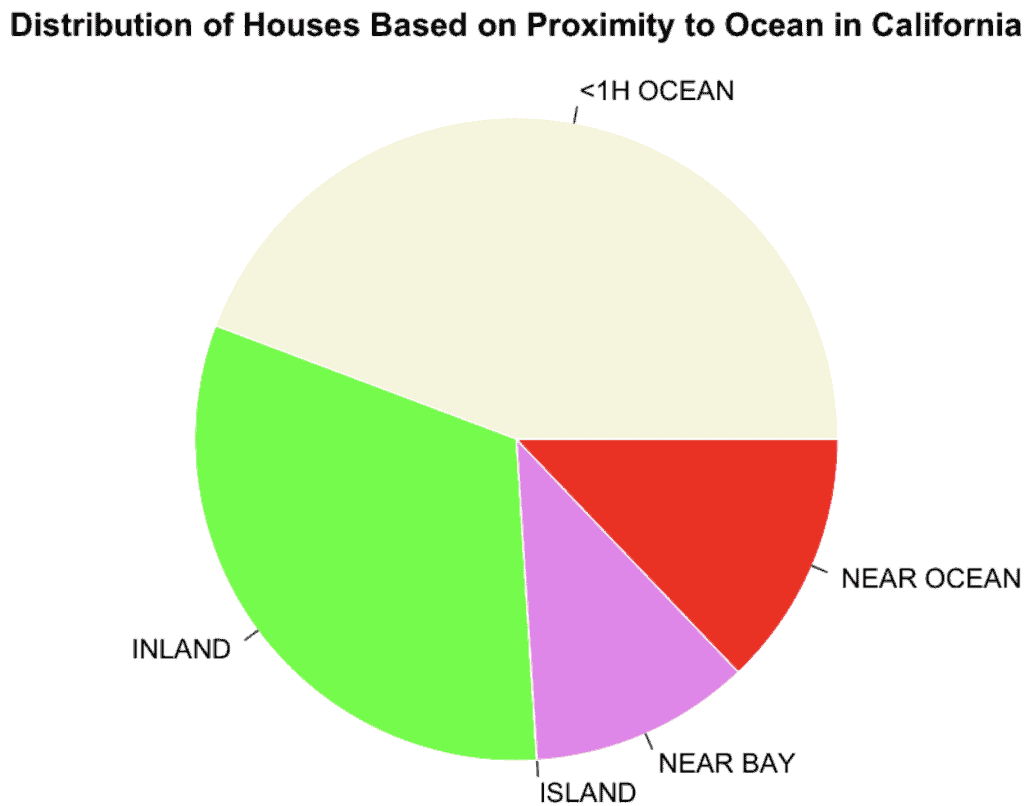You can see from the above chart image that we changed the title and color of the chart.

## Displaying Slice Percentages and Chart Legend

To add slice percentages to the pie chart created using the base R pie() function and display a legend, follow these steps:

### Calculate the percentages for each slice:

``````data_summary <- table(data\$ocean_proximity)

percentages <- round((data_summary / sum(data_summary)) * 100, 1)

labels <- paste(names(data_summary), "\n", percentages, "%")

colors <- c("<1H OCEAN" = "beige", "INLAND" = "green",
"NEAR OCEAN" = "blue", "NEAR BAY" = "violet", "ISLAND" = "red")``````

### Create a pie chart with the percentage

``````pie(data_summary,
labels = labels,
main="Distribution of Houses Based on Proximity to Ocean in California",
col=colors,
border="white",
``````

``legend("topright", legend = names(data_summary), fill = colors, cex = 0.8, bty = "n")``

### Complete code

``````data <- read_csv("DataSets/california_housing.csv")

data_summary <- table(data\$ocean_proximity)

percentages <- round((data_summary / sum(data_summary)) * 100, 1)

labels <- paste(names(data_summary), "\n", percentages, "%")

colors <- c("<1H OCEAN" = "beige", "INLAND" = "green",
"NEAR OCEAN" = "blue", "NEAR BAY" = "violet", "ISLAND" = "red")

pie(data_summary,
labels = labels,
main="Distribution of Houses Based on Proximity to Ocean in California",
col=colors,
border="white",

legend("topright", legend = names(data_summary), fill = colors, cex = 0.8, bty = "n")``````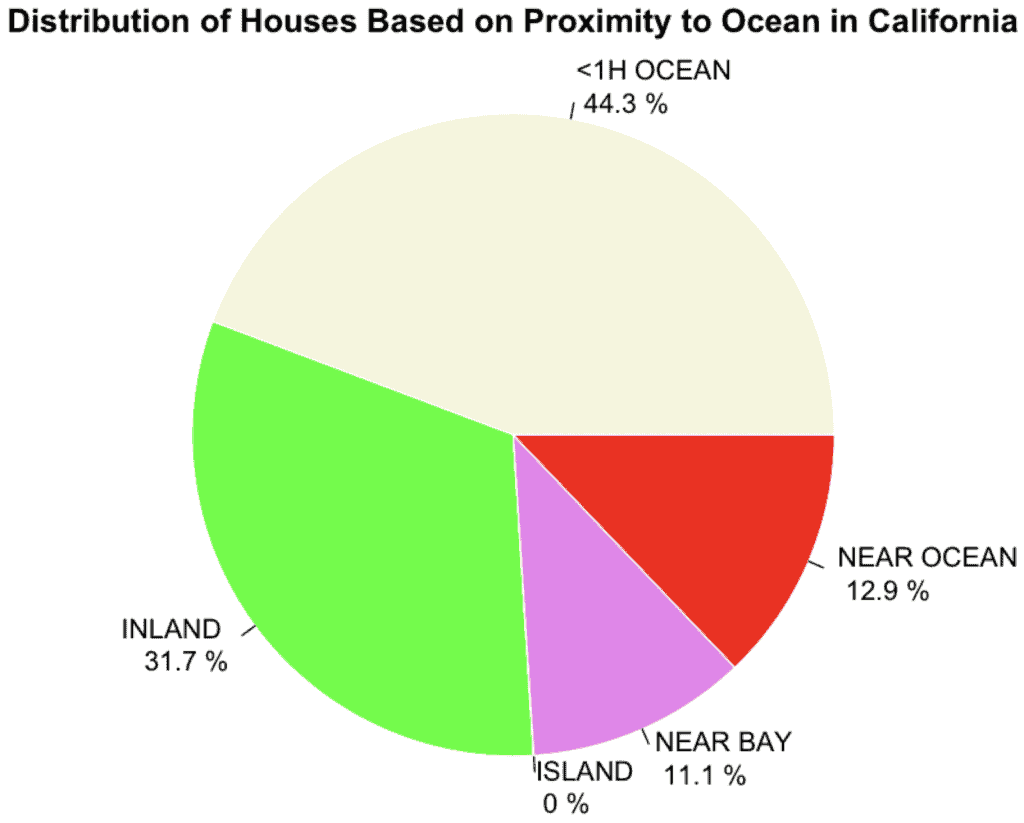You can see the slices percentage-wise, which can be helpful to analyze the data more accurately.

The legend() function is used to add a legend to the pie chart, positioned at the top right of the chart (“topright”).

The cex parameter is used to adjust the size of the legend, and bty = “n” removes the box around the legend.

## 3D Pie Chart

To create a 3D pie chart in R, use the “plotrix package’s pie3D() function”. We already installed the package at the start of this tutorial. We need to load the library using the library() method.

``library(plotrix)``

Now, write a code to construct a 3D chart.

``````library(plotrix)

# Compute the data for pie chart
data_summary <- data %>%
group_by(ocean_proximity) %>%
summarize(count = n())

# Custom colors
colors <- c("blue", "beige", "green", "red", "yellow")

# Create a 3D pie chart
pie3D(data_summary\$count, labels = data_summary\$ocean_proximity,
main="Distribution of Houses Based on Proximity to Ocean in California",
col=colors, explode=0.1, theta=1.2)``````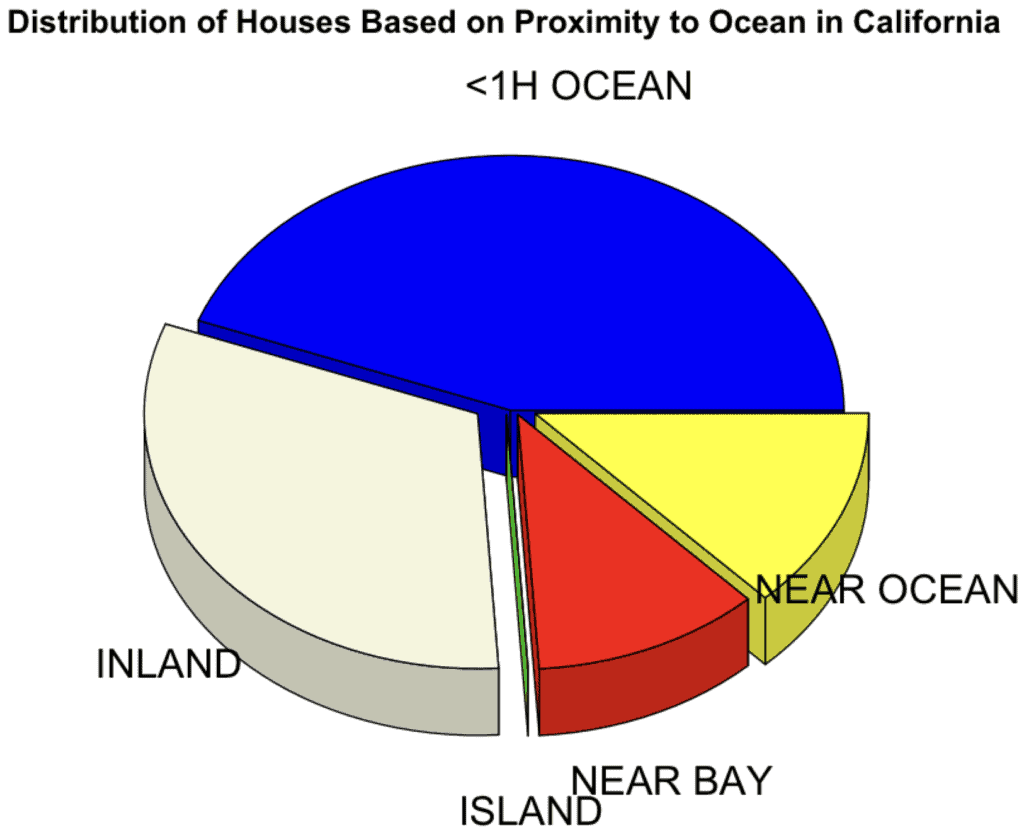The output image is somewhat distorted due to the overlapping of labels.

There are two points to note about the pie3D() function:

1. The explode parameter will separate the slices a bit for better visibility.
2. The theta parameter controls the angle of the viewing position, influencing the 3D appearance.

We created a 3D pie chart representing the distribution of the housing data based on ocean proximity.

Always ensure that your visualizations accurately represent the data and are easily interpretable.

I hope you understand how the pie chart works and what modifications you can make based on your project requirements. For more visual appeal, we created a 3D pie chart, which is fantastic.